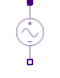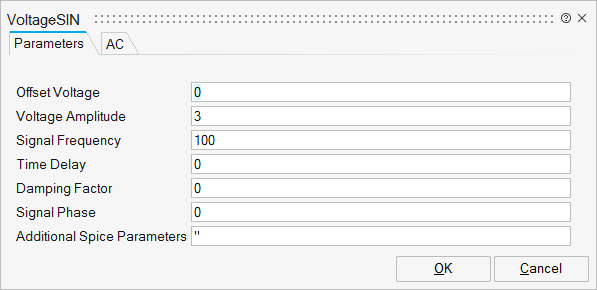# VoltageSIN

Time-dependent sine wave voltage source.Analog/Basic

## Description

The VoltageSin is a sinusoidal voltage source with a constant frequency.

The peak value or amplitude value is measured from zero (if the initial DC offset value is not set) or measured from the DC offset value (if given).

## ParametersNameLabelDescriptionData TypeValid Values

sp_SIN

SIN

String

sp_V0

Offset Voltage

The initial DC offset voltage (volts). Set to zero if you need a pure sinusoid.

Scalar

sp_V1

Voltage Amplitude

The undamped amplitude of the sinusoid, i.e. the peak value measured from zero or from the DC offset value.

Scalar

sp_Freq

Signal Frequency

The frequency of the sinusoid (Hertz)

Scalar

sp_Td

Time Delay

The time delay (seconds). Set to zero for the normal sinusoid.

Scalar

sp_Theta

Damping Factor

The damping factor. Set to zero for the normal sinusoid. This is used to apply an exponential decay to the sinusoid.

Scalar

sp_Phase

Signal Phase

The phase advance (degrees). Set to 90 if you need a cosine waveform.

Scalar

sp_Spice

For more details, see Additional Spice Parameters.

String

## Ports

NameTypeDescriptionIO TypeNumber

V+

implicit

Positive pin

1

V-

implicit

Negative pin

2